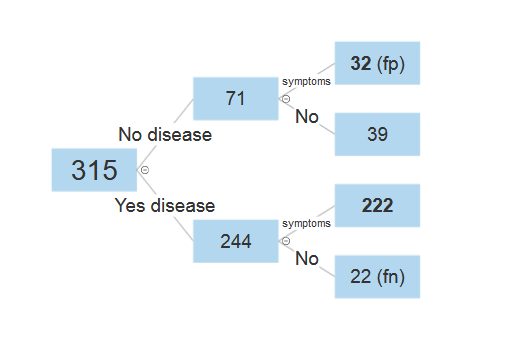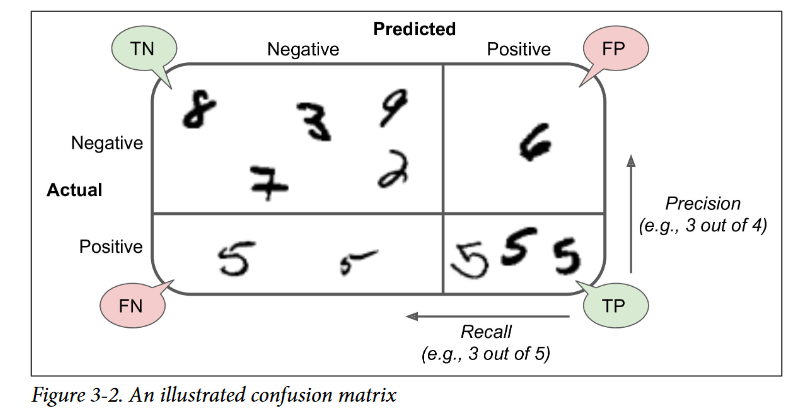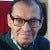# Bayes Theorem & Confusion Matrix

Bayes’ theorem is crucial for interpreting the results from binary classification algorithms.

We will show that Bayes’ theorem is simply the relationship between precision and recall (precisely it is the precision), then we can turn the process into an equation, which is Bayes’ Theorem. It lets you take the test results and correct for the “skew” introduced by false positives. Let me show that with some real numbers of a confusion matrix.

print(confusion_matrix(y_test, y_pred))
print(classification_report(y_test, y_pred))

dtype: int64
[[ 39 32] 71 = A = 0
[ 22 222]] 244 =B = 1
254 315

`___________Predict Actual 0 [[ 39 32] 71 Actual 1 [ 22 222]] 244 ___________61 254 315`

1. precision: 222/254 =0.874 P(A/B)
2. recall: 222/244 =0.909 = Bayes P(B/A)

So the recall (sensitivity) results in Bayes: P(B/A)

The probability P(B/A) = 222/244 = 0.91 is called the recall. It simply gives the percentage of the 244 actual B that were classified by our classification algorithm. We can see (maybe not at first glance) that Bayes’ theorem is simply a relationship between recall and precision:

[Predict * precision / Actual = recall] = Bayes

(P_B*P_AB)/P_A = 0.9098360655737705

(P(B)*P(A/B))/(P(A)) = 0.9098360655737705 = P(B/A)
(254/315 * 222/254) / (244/315) = 0.9098
predict * precision / actual = recall

And what is the question again? Oh really yes: what’s the chance we really have an illness or disease if we get a positive result in our case. This is a bit like the dog of two head (recall or precision). The answer is 87 % (this precision as specificity):

`Bayes Theoremr1 Actual_probability = 77%                      = 0.77 r2 Prob_true_positive = 90%                      = 0.9 r3 Prob_false_positive = 45%                     = 0.45 Chance positive test means positive result                                             >>>\$(r1 * r2)/((r1 * r2) + r3*(1-r1)) * 100      = 87.01  %---https://instacalc.com/52323`

r1 is actual, r2 is recall, r3 is 32/71 = 45% as Prob_false_positive

https://instacalc.com/52323

I transform the formula above to predict:

predict = actual * recall / precision = ~80% = 77 * 90 / 87
or precision = actual * recall / predict = 87%

Lets compare with the Bayes formula

prior * likelihood / evidence = posterior
actual * recall / predict = precision

prior -> actual; likelihood -> recall; evidence -> predict

`precision recall f1-score support 0 0.64 0.55 0.59 71 1 0.87 0.91 0.89 244`

`accuracy 0.83 315`

`macro avg 0.76 0.73 0.74 315`

`weighted avg 0.82 0.83 0.82 315`

Now the proof of concept with a decision tree; you may be able to immediately see that to answer this question with a simple ratio: number of diseased people with symptoms / total number of people with symptoms (includes false positive). What is the probability a patient with symptoms actually has the disease?

Number of people with disease and symptoms (222) / total number with symptoms (222 + 32)

which gives us: 222 / 254 = 0.87 = 87.4%.
Now let’s construct the same answer with a probability tree:

Which is exactly the same answer you would get by actually working the formula 87%. I’ve never come across a Bayes’ related problem that can’t be answered with a probability (decision) tree!Bayes Theorem Formula with Decision Tree

Bayes matrix detector compute ends…, Confusion matrix needs both labels & predictions as single-digits, not as one-hot encoded vectors; although you have done this with your predictions using model.predict_classes()

<iframe src=”https://instacalc.com/54440/embed" width=”450" height=”350" frameborder=”0"></iframe>

You can explore that more with a forked jupyter notebook in python: https://github.com/maxkleiner/Bayes_theorem/blob/master/Bayes_Theorem.ipynb

http://www.softwareschule.ch/examples/bayes_matrix.htmFrom Screenshot_2021–05–02 Hands-On Machine Learning with Scikit-Learn and TensorFlow Book.

--

--## Max Kleiner

Max Kleiner's professional environment is in the areas of OOP, UML and coding - among other things as a trainer, developer and consultant.